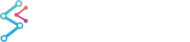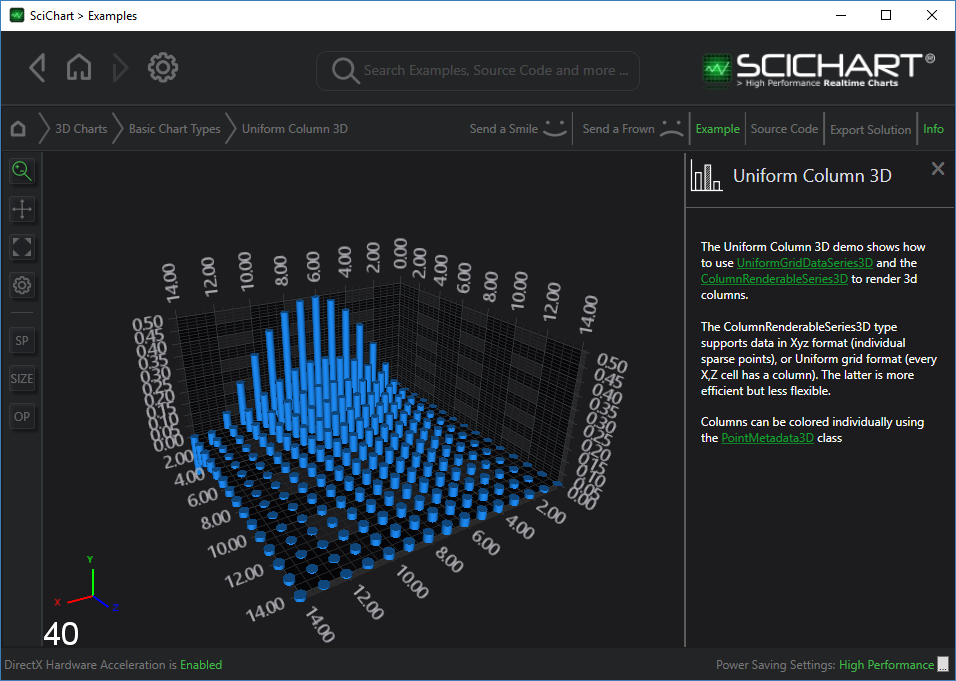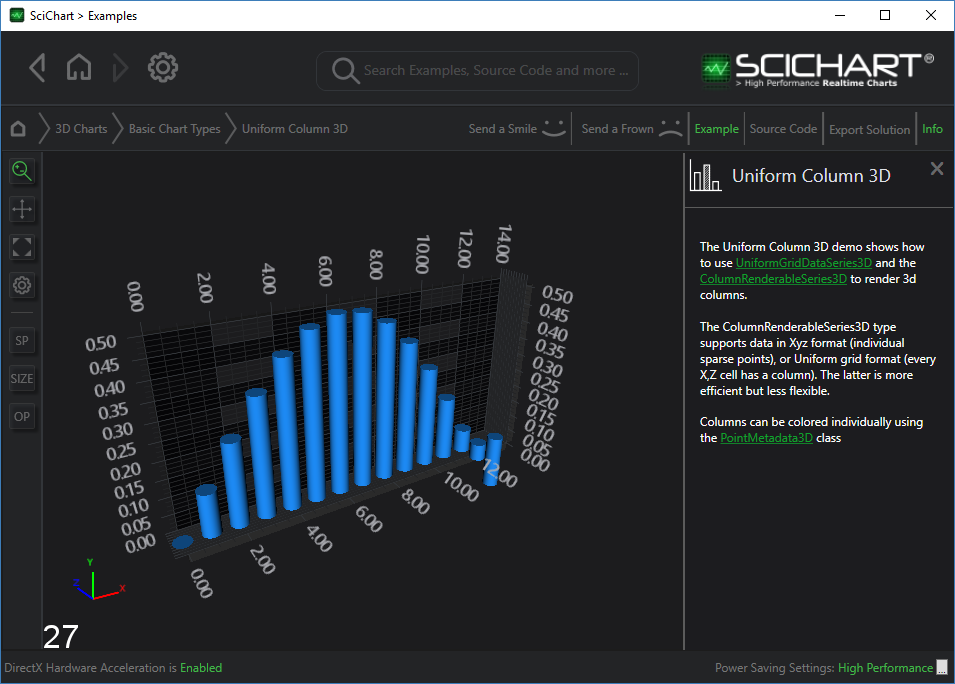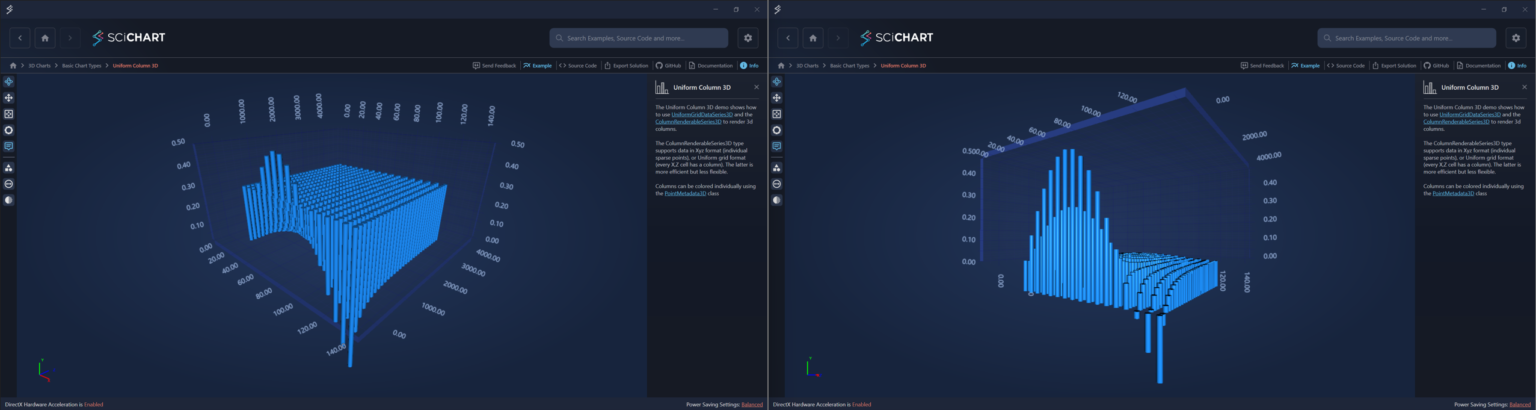﻿ The Column 3D Chart Type | WPF Chart DocumentationSciChart WPF 3D Charts > 3D Chart Types > The Column 3D Chart Type
The Column 3D Chart Type

3D Column Charts are provided by the ColumnRenderableSeries3D type.## Declaring a 3D Column Chart

The ColumnRenderableSeries3D accepts either XyzDataSeries3D for sparse points, Or UniformGridDataSeries3D for an NxM array of points.

The above graph is rendered with the following code:

```<s3D:SciChart3DSurface x:Name="SciChart"
BorderThickness="0"
WorldDimensions="200,100,200">
<s3D:SciChart3DSurface.RenderableSeries>
<!--  To create a Scatter Chart, create a ScatterRenderableSeries3D and use a 3D point marker type  -->
<s3D:ColumnRenderableSeries3D ColumnShape="{x:Type s3D:CylinderPointMarker3D}"
DataPointWidthX="0.5"
Opacity="1"/>
</s3D:SciChart3DSurface.RenderableSeries>
<s3D:SciChart3DSurface.XAxis>
<s3D:NumericAxis3D />
</s3D:SciChart3DSurface.XAxis>
<s3D:SciChart3DSurface.YAxis>
<s3D:NumericAxis3D VisibleRange="0,0.5" />
</s3D:SciChart3DSurface.YAxis>
<s3D:SciChart3DSurface.ZAxis>
<s3D:NumericAxis3D />
</s3D:SciChart3DSurface.ZAxis>
</s3D:SciChart3DSurface>
```
```private void OnLoaded(object sender, RoutedEventArgs routedEventArgs)
{
const int Count = 15;
var uniformDataSeries = new UniformGridDataSeries3D<double>(Count, Count)
{
StepX = 1,
StepZ = 1,
SeriesName = "Column 3D Data",
};
for (var x = 0; x < Count; x++)
{
for (var z = 0; z < Count; z++)
{
var y = Math.Sin(x * 0.25) / ((z + 1) * 2);
uniformDataSeries[z, x] = y;
}
}
SciChart.RenderableSeries.DataSeries = uniformDataSeries;
}
```

However it could be just as easily created with an XyzDataSeries3D as the DataSeries for sparse columns.

## Single Row Column 3D Charts

By changing a few parameters, it is possible to get a column chart to look like this:The code to achieve the above is as follows:

```<s3D:SciChart3DSurface x:Name="SciChart"
BorderThickness="0"
WorldDimensions="200,100,200">
<s3D:SciChart3DSurface.RenderableSeries>
<!--  To create a Scatter Chart, create a ScatterRenderableSeries3D and use a 3D point marker type  -->
<s3D:ColumnRenderableSeries3D ColumnShape="{x:Type s3D:CylinderPointMarker3D}"
DataPointWidthX="0.05"
Opacity="1"/>
</s3D:SciChart3DSurface.RenderableSeries>
<s3D:SciChart3DSurface.XAxis>
<s3D:NumericAxis3D />
</s3D:SciChart3DSurface.XAxis>
<s3D:SciChart3DSurface.YAxis>
<s3D:NumericAxis3D VisibleRange="0,0.5" />
</s3D:SciChart3DSurface.YAxis>
<s3D:SciChart3DSurface.ZAxis>
<s3D:NumericAxis3D DrawLabels="False"/>
</s3D:SciChart3DSurface.ZAxis>
</s3D:SciChart3DSurface>
```
```private void OnLoaded(object sender, RoutedEventArgs routedEventArgs)
{
const int Count = 15;
var uniformDataSeries = new UniformGridDataSeries3D<double>(Count, 1)
{
StepX = 1,
StepZ = 1,
SeriesName = "Column 3D Data",
};
for (var x = 0; x < Count; x++)
{
for (var z = 0; z < 1; z++)
{
var y = Math.Sin(x * 0.25) / ((z + 1) * 2);
uniformDataSeries[z, x] = y;
}
}

SciChart.RenderableSeries.DataSeries = uniformDataSeries;
}
```

## 3D Column Shapes

The column shape can be defined by one of several pointmarkers, including:

Apply the Type of point marker to the ColumnRenderableSeries3D.ColumnShape property to change the column shape.

## Setting Column Sizes

Column sizes are set as a ratio of the available space between the neighbouring points.

Setting the properties ColumnRenderableSeries3D.DataPointWidthDataPointWidthXDataPointWidthZ changes the ratio of space allocated to the column in the X & Z direction.

## ZeroLineY for 3D Columns

This feature allows specifying Zero position for 3D Column series relatively to YAxis. By default, all 3D Columns begin at XZ plane position. Now it is possible to set Y-Value at which 3D Columns will start:This can be achieved setting ZeroLineY property on ColumnRenderableSeries3D instance:

Setting ZeroLineY property
Copy Code
```<s3D:SciChart3DSurface.RenderableSeries>
<!--  To create a 3D Columns Chart, create a ColumnRenderableSeries3D. Optionally specify ZeroLineY, ColumnShape, Opacity  -->
<s3D:ColumnRenderableSeries3D ZeroLineY="-0.15"
DataPointWidthX="0.8"
Opacity="1"/>
</s3D:SciChart3DSurface.RenderableSeries>
```